# Heptagonal pyramid

A hardwood for a column is in the form of a frustum of a regular heptagonal pyramid. The lower base edge is 18 cm and the upper base of 14 cm. The altitude is 30 cm. Determine the weight in kg if the density of the wood is 10 grams/cm3.

Correct result:

m =  17.3972 kg

#### Solution: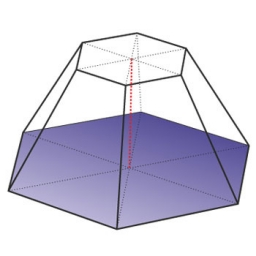We would be pleased if you find an error in the word problem, spelling mistakes, or inaccuracies and send it to us. Thank you!Tips to related online calculators
Looking for a statistical calculator?
Do you know the volume and unit volume, and want to convert volume units?
Tip: Our Density units converter will help you with the conversion of density units.
Do you want to convert mass units?
Most natural application of trigonometry and trigonometric functions is a calculation of the triangles. Common and less common calculations of different types of triangles offers our triangle calculator. Word trigonometry comes from Greek and literally means triangle calculation.

#### You need to know the following knowledge to solve this word math problem:

We encourage you to watch this tutorial video on this math problem:

## Next similar math problems:

• Tetrahedral pyramidDetermine the surface of a regular tetrahedral pyramid when its volume is V = 120 and the angle of the sidewall with the base plane is α = 42° 30´.
• Octagonal pyramidFind the volume of a regular octagonal pyramid with height v = 100 and the angle of the side edge with the plane of the base is α = 60°.
• Paper box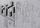Calculate the consumption of paper on the box-shaped quadrangular prism with rhombic footstall, base edge a=6 cm and the adjacent base edges forms an angle alpha = 60 °. Box height is 10 cm. How many m2 of the paper consumed 100 such boxes?
• Digging a pit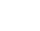The pit has the shape of a regular quadrilateral truncated pyramid. The edges of the bases are 14m and 10m long. The sidewalls form an angle of 135° with a smaller base. Determine how many m3 of soil were excavated when digging the pit?
• FlowerbedFlowerbed has the shape of a truncated pyramid, the bottom edge of the base a = 10 m, the upper base b = 9 m. Deviation angle between edge and the base is alpha = 45°. What volume is needed to make this flowerbed? How many plants can be planted if 1 m2 =
• 4side pyramidCalculate the volume and surface of 4 sides regular pyramid whose base edge is 4 cm long. The angle from the plane of the sidewall and base plane is 60 degrees.
• House roofThe roof of the house has the shape of a regular quadrangular pyramid with a base edge 17 m. How many m2 is needed to cover roof if roof pitch is 57° and we calculate 11% of waste, connections and overlapping of area roof?
• Sphere in coneA sphere is inscribed in the cone (the intersection of their boundaries consists of a circle and one point). The ratio of the surface of the ball and the contents of the base is 4: 3. A plane passing through the axis of a cone cuts the cone in an isoscele
• Tetrahedral pyramidCalculate the volume and surface of the regular tetrahedral pyramid if content area of the base is 20 cm2 and deviation angle of the side edges from the plane of the base is 60 degrees.
• Decagon prism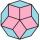A regular decagon of side a = 2 cm is the base of the perpendicular prism, the side walls are squares. Find the prism volume in cm3, round to two decimal places.
• Pillar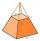Calculate volume of pillar shape of a regular tetrahedral truncated pyramid, if his square have sides a = 19, b = 27 and height is h = 48.
• Roof 8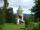How many liters of air are under the roof of tower which has the shape of a regular six-sided pyramid with a 3,6-meter-long bottom edge and a 2,5-meter height? Calculate the supporting columns occupy about 7% of the volume under the roof.
• GlassHow many glass are needed to produce glass with base regular 5-gon if one base triangle in the base is 4.2 square cm and the height is 10 cm?
• Pentagonal prismThe regular pentagonal prism is 10 cm high. The radius of the circle of the described base is 8 cm. Calculate the volume and surface area of the prism.
• Pyramid 8Calculate the volume and the surface area of a regular quadrangular pyramid with the base side 9 cm and side wall with the base has an angle 75°.
• Tetrahedral pyramid 8Let’s all side edges of the tetrahedral pyramid ABCDV be equally long and its base let’s be a rectangle. Determine its volume if you know the deviations A=40° B=70° of the planes of adjacent sidewalls and the plane of the base and the height h=16 of the p
• Triangular prismPlane passing through the edge AB and the center of segmet CC' of regular triangular prism ABCA'B'C', has angle with base 22 degrees, |AB| = 6 cm. Calculate the volume of the prism.